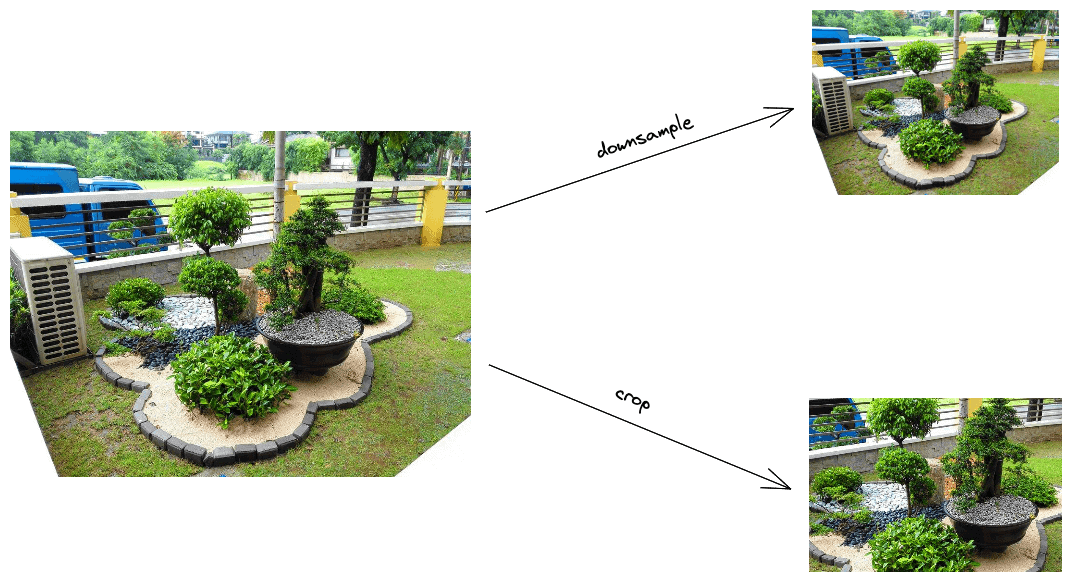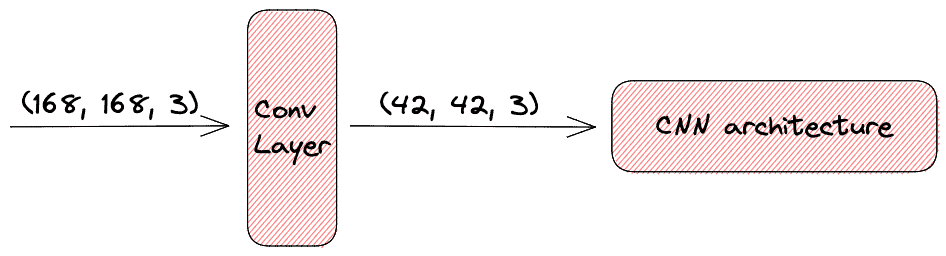## 1. Overview

In this tutorial, we’ll talk about how to handle large images to train Convolutional Neural Networks (CNNs). First, we’ll introduce CNNs and the difficulties of using large images as input to CNNs. Then, we’ll describe three ways to handle large images: resize the image, increase the model size or process the images in batches.

## 2. Introduction to CNNs

One of the most successful architectures in deep learning is the Convolutional Neural Networks (CNNs) which consists of one or more convolutional layers followed by some feed-forward layers. In a previous article, we discussed the differences between these types of layers.

CNNs are primarily used in visual tasks like image classification, image segmentation, and reconstruction. However, during the last years, CNNs are also employed in other tasks like speech recognition and natural language processing. In this article, we’ll focus on the use of CNNs in visual tasks where the input of the model is an image.

## 3. The Problem of Large Images

A crucial characteristic of CNNs is the fact the input image should be fixed in size.

Specifically, the total number of parameters in a CNN depends on the input size. During training, the total number of parameters should be stable. That’s why the input size should be stable as well.

Also, a common practice in computer vision is to use already trained CNNs for pretraining. This practice facilitates the training of a model since we use some prior knowledge about the image distribution we want to learn. However, the pretrained CNNs have been trained using a specific input size.

We can easily understand that the fixed input size creates a problem in training CNNs since we are restricted to using a predefined input size that may not be equal to the size of our images. Below, we will discuss three solutions for using large images in CNN architectures that take as input smaller images.

## 4. Resize

One solution is to resize the input image so that it has the same size as the required input size of the CNN. There are many ways to resize an input image. In this article, we’ll focus on two of them.

### 4.1. Downsample the Image

When we downsample an image, our goal is to reduce the spatial resolution of the image while keeping the same two-dimensional representation. The simplest way to downsample an image is to skip some pixels. For example, in order to reduce an image to half of its size, we can skip one every two pixels.

However, the well-known problem of aliasing appears in this case, where high-frequency changes (like changing light and dark colors) will convert to low-frequency changes (like constant dark and light).

A better downsampling technique that prevents aliasing is averaging, where we averagepixels into a single pixel.

### 4.2. Crop the Image

If we don’t want to keep the whole content of the image, we can reduce its size by cropping down the image to the size we want. Usually, we crop the image from the center part so as to keep the central content that is usually more useful and significant for the image.

In the diagram below, we can see an example of using the above methods:## 5. Increase Model Size

Until now, we dealt with the problem of large input images to CNNs by reducing the size of the input image. Another possible way could be to keep the size of the input stable and modify the CNN architecture accordingly. Specifically, we can make use of the fact that convolutional layers can reduce the size of an input image. So, we can add some additional convolutional layers before the convolutional layers of the CNN architecture and end up with an architecture that can handle larger images.

In the image below, we can see an example of using a large image to a CNN by adding an extra convolutional layer. Specifically, the convolutional layer decreases the input size by a factor of 4:## 6. Process Images in Batches

The third solution to our problem is to process the large input images in batches and reduce the overall memory load. Specifically, a problem when using large images is that the whole training set can’t fit into our memory. The solution comes with Mini-Batch Gradient Descent, where we iterate through the dataset and process a group of images each time. So, we can adjust the size of this group (batch) to fit the large images into our memory.

## 7. Conclusion

In this article, we presented three ways of using large images as input to CNNs. First, we introduced the problem of using large images to these architectures, and then we described the three solutions along with detailed examples.